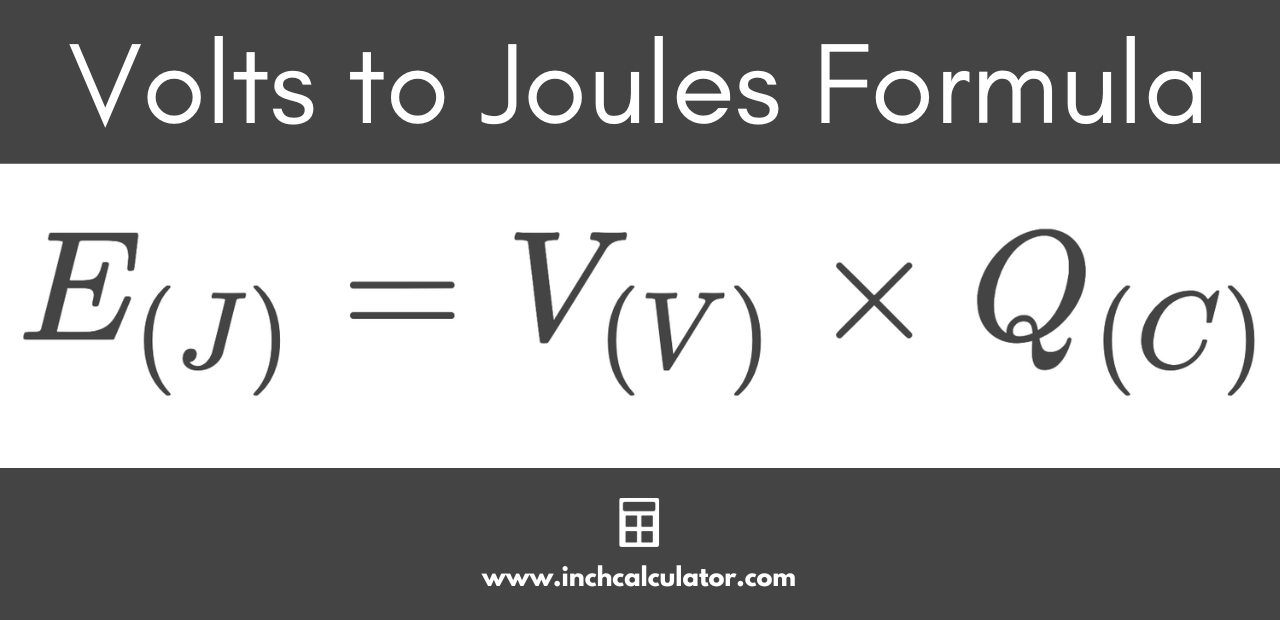# Volts to Joules Conversion Calculator

Convert volts to joules by entering the voltage and the charge in coulombs below.

V
C

## Joules Results:

J
Learn how we calculated this below

## How to Convert Volts to Joules

Volts are a measure of voltage while coulombs measure electric charge. Together, these measurements can be used to find the energy in joules using a formula.

### Volts to Joules Formula

The formula to convert volts to joules is:

E(J) = V(V) × Q(C)

Thus, the energy E in joules is equal to the voltage V in volts times the charge Q in coulombs.For example, let’s convert a charge of 4 coulombs at 12 volts to joules.

E(J) = 12 V × 4 C
E(J) = 48 J

So, a charge of 4 coulombs at 12 volts is equal to 48 joules of energy.

You should also try our watts to joules calculator.